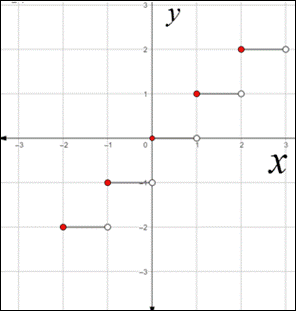# To sketch the graph of the function### Precalculus: Mathematics for Calcu...

6th Edition
Stewart + 5 others
Publisher: Cengage Learning
ISBN: 9780840068071### Precalculus: Mathematics for Calcu...

6th Edition
Stewart + 5 others
Publisher: Cengage Learning
ISBN: 9780840068071

#### Solutions

Chapter 2.5, Problem 68E
To determine

## To sketch the graph of the function

Expert Solution

### Explanation of Solution

Given information:

The function is y=x

Concept used:

The greatest integer function is defined by,

x is the greatest integer less than or equal to x .

The horizontal shrinking and stretching of graph are defined by,

To graph, y=f(cx) ;

If c>1 , shrink the graph of y=f(x) horizontally by a factor of 1c .

If 0<c<1 , stretch the graph of y=f(x) horizontally by a factor of 1c .

Graph:

To sketch the graph of the function f(x)=x , substitute different value of x and find the corresponding value of f(x)=x .

The table showing the values of f for some value of x is given below,

 x y=〚x〛 ⋮ ⋮ −2≤x<−1 −2 −1≤x<0 −1 0≤x<1 0 1≤x<2 1 2≤x<3 2 ⋮ ⋮

The function is constant between consecutive integers, so the graph between integers is a horizontal line segment as shown below:To graph y=14x , use horizontal shrinking and stretching of graph,

Since, 0<c=14<1 , so, the graph of y=14x is obtained by stretching the graph of y=x horizontally by a factor of 14 .

Hence,

The graph of y=14x is obtained as:Interpretation:

From the above graph, it can be observed that graph of y=14x is obtained by stretching the graph of y=x horizontally by a factor of 14 .

### Have a homework question?

Subscribe to bartleby learn! Ask subject matter experts 30 homework questions each month. Plus, you’ll have access to millions of step-by-step textbook answers!